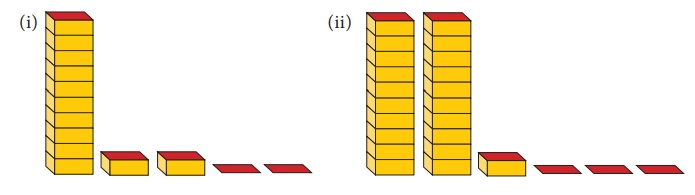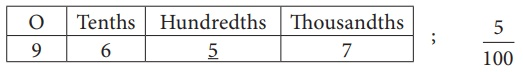Home | | Maths 7th Std | Exercise 1.1

# Exercise 1.1

7th Maths : Term 2 Unit 1 : Number System : Exercise 1.1 : Text Book Back Exercises Questions with Answers, Solution

Exercise 1.1

1. Write the decimal numbers for the following pictorial representation of numbers.(i) 12.2

(ii) 21.3

2. Express the following in cm using decimals.

(i) 5 mm

(ii) 9 mm

(iii) 42 mm

(iv) 8 cm 9 mm

(v) 375 mm

i) 5 mm = 5/10 cm = 0.5 cm

ii) 9 mm = 9/10 cm = 0.9 cm

iii) 42 mm = 42/10 cm = 4.2 cm

iv) 8 cm 9 mm = 8 +   42/10 cm  = 8.9 cm

v) 375 mm = 375/10 cm = 37.5 cm

3. Express the following in metres using decimals.

(i) 16 cm

(ii) 7 cm

(iii) 43 cm

(iv) 6 m 6 cm

(v) 2 m 54 cm

i) 16 cm = 16 /100 m = 0.16 m

ii) 7 cm = 7/100 m = 0. 07m

iii) 43 cm = 43 /100 m = 0.43 m

iv) 6 m 6 cm = 6 +   6 /100 m = 6.06 m

v) 2 m 54 cm = 2 +   54/100 m = 2.54 m

4. Expand the following decimal numbers.

(i) 37.3

(ii) 658.37

(iii) 237.6

(iv) 5678.358

i) 37.3

Expanded form of

37.3 = 30 + 7 +  (3/10)

ii) 658.37

Expanded form of

65837 = 600 + 50 + 8 +  3/10 + 7/100

iii) 237.6

Expanded form of

2376= 200 + 30 + 7+  6/10

iv) 5678.358

Expanded form of

5678358 = 5000 – 600 + 70 + 8 + 3/10 + 5/100 + 8 /1000

5. Express the following decimal numbers in place value grid and write the place value of the underlined digit.

(i) 53.61

(ii) 263.271

(iii) 17.39

(iv) 9.657

(v) 4972.068

(i) 53.61

Place value grid53.6 1

The place value of 6 in 53.61 is Tenth.

(ii) 263.2 71

Place value grid263.2 71

The place value of 2 in 263.271 is Tenth = 2/10

(iii) 17.39

Place value grid17.39

The place value of 9 in 17.39 is Hundredth = 9/100

(iv) 9.657

Place value grid9.657

The place value of 5 in 9.657 is Hundredth = 5/100

(v) 4972.068

Place value grid4972.068

The place value of 8 in 4972.068 is Thousandth = 8/1000

Objective type questions

6. The place value of 3 in 85.073 is _______

(i) tenths

(ii) hundredths

(iii) thousands

(iv) thousandths

7. To convert grams into kilograms, we have to divide it by

(i) 10000

(ii) 1000

(iii) 100

(iv) 10

8. The decimal representation of 30 kg and 43 g is ____ kg.

(i) 30.43

(ii) 30.430

(iii) 30.043

(iv) 30.0043

9. A cricket pitch is about 264 cm wide. It is equal to ____ m.

(i) 26.4

(ii) 2.64

(iii) 0.264

(iv) 0.0264

Exercise 1.1

1. (i) 12.2 (ii) 21.3

2. (i) 0.5 cm (ii) 0.9 cm (iii) 4.2 cm (iv) 8.9 cm (v) 37.5 cm

3. (i) 0.16 m (ii) 0.07 m (iii) 0.43 m (iv) 6.06 m (v) 2.54 m

4. (i) 30 + 7 + 3/10 (ii) 600 + 50 + 8 + 3/10 + 7/100 (iii) 200+30+7+  6/10 (iv) 5000 + 600 + 70 + 8 + 3/10 + 5/100 + 8/1000

5.Objective type questions

6. (iv) thousandths

7. (ii) 1000

8. (iii) 30.043

9. (ii) 2.64

Tags : Questions with Answers, Solution | Number System | Term 2 Chapter 1 | 7th Maths , 7th Maths : Term 2 Unit 1 : Number System
Study Material, Lecturing Notes, Assignment, Reference, Wiki description explanation, brief detail
7th Maths : Term 2 Unit 1 : Number System : Exercise 1.1 | Questions with Answers, Solution | Number System | Term 2 Chapter 1 | 7th Maths